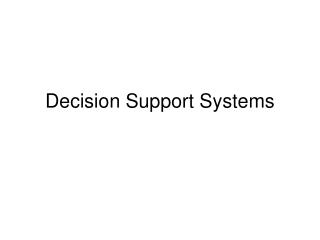DownloadDownload PresentationDecision Support Systems

# Decision Support Systems

Télécharger la présentation## Decision Support Systems

- - - - - - - - - - - - - - - - - - - - - - - - - - - E N D - - - - - - - - - - - - - - - - - - - - - - - - - - -
##### Presentation Transcript

1. Decision Support Systems

2. Rule based Systems if A then B • If pump failure then the pressure is low • If pump failure then check oil level • If power failure then pump failure Uncertainty If A (with certainty x) then B (with certainty f(x)) If C (with certainty x) then B (with certainty g(x) If we now get the information that A holds with certainty a and C holds with certainty c, what is the certainty of B?

3. Rule based Systems – cont. • Ifblood glucose is low before lunch, then take less insulin in the morning • Model of the doctor • Easy to build ? • Easy to maintain ? • Easy to understand for clinicians and patients ? • Problems with uncertainty and variability

4. Rule based Systems – cont.

5. Neural Networks

6. Bayesian Networks • If tonsillitis then P(temp>37.9) = 0.75 • If whooping cough then P(temp>37.9) = 0.65One could be lead to read this as rules. They shouldn't be. So a different notation is used: • P(temp>37.9 | whooping cough) = 0.65 • P(temp>37.9 | whooping cough, tonsillitis)

7. Bayesian Networks - cont Bayes' theorem: P(A | B)P(B) = P(B | A)P(A) • uses a global perspective • calculates the new probabilities correctly • in rule based systems you try to model the experts way of reasoning (hence the name expert systems), while with Bayesian networks you try to model dependencies in the domain itself

8. Example diabetes • Predictions of blood glucose levels based on mathematical models of the carbohydrate metabolism • Illustrate the effect of changing e.g. insulin • Model of the patient • Can handle uncertainty and variability ? • Problems with various factors, e.g. stress, fever, alcohol, exercise etc.

9. Comparing Neural Networks and Bayesian Networks The fundamental difference between the two types of networks is that a perceptrone in the hidden layers does not in itself have an interpretation in the domain of the system, whereas all the nodes of a Bayesian network represent concepts that are well defined with respect to the domain.